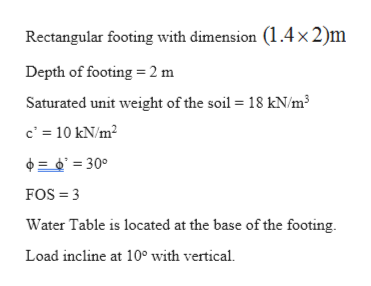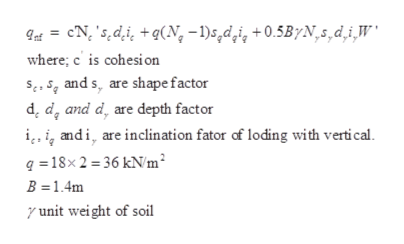# A rectangular footing, with a plan area of 1-4mx2·0m is to be placed 2m below the ground surface. The footing would be subjected to a load inclined at 10° to the vertical. The subsoil is clayey, sandy silt with saturated unit weight of 18 kN /m3, and c' = 10kN/m2 and cp' = 30°. Assuming the rate of loading is such that drained condition prevailed, compute the magnitude of load carrying capacity of the footing if. the water table is at the base of the footing. Use IS: 6403-1981 recommendation and assume factor of safety= 3·0.

Question
8 views

A rectangular footing, with a plan area of
1-4mx2·0m is to be placed 2m below the ground
surface. The footing would be subjected to a load
inclined at 10° to the vertical. The subsoil is clayey,
sandy silt with saturated unit weight of 18 kN /m3, and
c' = 10kN/m2 and cp' = 30°. Assuming the rate of
compute the magnitude of load carrying capacity of
the footing if. the water table is at the base of the
footing. Use IS: 6403-1981 recommendation and
assume factor of safety= 3·0.

check_circle

Step 1

Given Information:help_outlineImage TranscriptioncloseRectangular footing with dimension (1.4× 2)m Depth of footing = 2 m Saturated unit weight of the soil = 18 kN/m3 c' = 10 kN/m? 0 = 0' = 30° FOS = 3 Water Table is located at the base of the footing. Load incline at 10° with vertical. fullscreen
Step 2

Type of failure:

Since ϕ = 30o (local shear failure will occur)

Step 3

Formula used for calculation of u...help_outlineImage Transcriptionclose9at = cN, 's,d,i, + q(N, -1)s,d,i, +0.5B/N,s,d,i,W' where; c' is cohesi on S,, 5q and s, are shape factor d, d, and d, are depth factor i̟, i, andi, are inclination fator of loding with vertical. q =18× 2 = 36 kN/m² B = 1.4m y unit wei ght of soil fullscreen

### Want to see the full answer?

See Solution

#### Want to see this answer and more?

Solutions are written by subject experts who are available 24/7. Questions are typically answered within 1 hour.*

See Solution
*Response times may vary by subject and question.
Tagged in

### Geotechnical Engineering